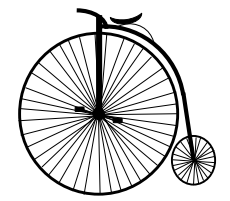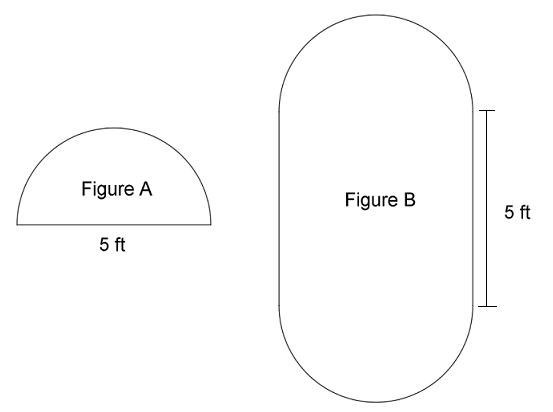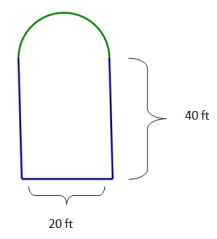# Geometry

## Objective

Solve real-world and mathematical problems using the relationship between the circumference of a circle and its diameter.

## Common Core Standards

### Core Standards

?

• 7.G.B.4 — Know the formulas for the area and circumference of a circle and use them to solve problems; give an informal derivation of the relationship between the circumference and area of a circle.

?

• 4.MD.A.3

## Criteria for Success

?

1. Know the formula that relates the circumference and diameter of a circle: ${{C=\pi d}.}$
2. Use the formula ${C=\pi d}$ to solve problems.
3. Understand that the distance around a closed semi-circle is half of the circumference added to the diameter of the circle.

## Tips for Teachers

?

Lessons 6 and 7 focus on the relationship between a circle’s circumference and its diameter. In Lesson 7, students solve real-world and mathematical problems involving circumference, including problems involving semi-circles.

#### Fishtank Plus

• Problem Set
• Student Handout Editor
• Vocabulary Package

## Anchor Problems

?

### Problem 1

Basil saw a strange old bicycle at the museum. It had one very big wheel and one very small one.

At home, Basil looked it up on the Internet and found that the big wheel could have a 52-inch diameter and the small wheel could have an 18-inch diameter.1. What is the circumference of the big wheel?
2. How far would you travel in one turn of the big wheel? Give your answer in feet and inches.
3. How many times must the cyclist turn the big wheel to travel 1 mile? A mile is 1,760 yards. Give your answer to the nearest 10 turns.
4. How many times does the small wheel turn when the cycle travels 1 mile?

#### References

MARS Summative Assessment Tasks for Middle School Historic Bicycle

Historic Bicycle from the Summative Assessment Tasks for Middle School is made available through the Mathematics Assessment Project under the CC BY-NC-ND 3.0 license. Copyright © 2007-2015 Mathematics Assessment Resource Service, University of Nottingham. Accessed March 10, 2018, 11:11 a.m..

### Problem 2

Two figures are shown below. Figure A is a semi-circle, and Figure B is composed of a square and two semi-circles.

Find the distance around each figure.## Problem Set

?The following resources include problems and activities aligned to the objective of the lesson that can be used to create your own problem set.

?

Briana’s parents built a swimming pool in the backyard in the shape shown below.

What is the distance around the pool?#### References

EngageNY Mathematics Grade 7 Mathematics > Module 3 > Topic C > Lesson 16Exit Ticket

Grade 7 Mathematics > Module 3 > Topic C > Lesson 16 of the New York State Common Core Mathematics Curriculum from EngageNY and Great Minds. © 2015 Great Minds. Licensed by EngageNY of the New York State Education Department under the CC BY-NC-SA 3.0 US license. Accessed Dec. 2, 2016, 5:15 p.m..

Modified by The Match Foundation, Inc.

?